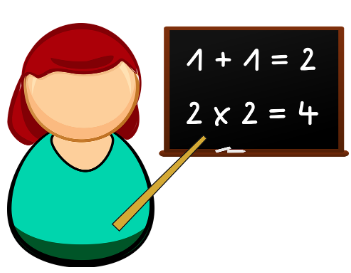# When Math Happens - 3 Act Math

Creative Communicator### Overview

Math can be solved through story problems. Most stories are divided into three acts.

3 Act math is a presentation of a math video and is completed in three parts.

• Act 1: Students watch a short video. They then make a hypothesis with a provided math prompt.
• Act 2: Information is given to help solve the math task. Students calculate the answer.
• Act 3: There is a final video with explanation and a short sequel.

Learn about how Dan Meyer and his ideas are helpful in solving mathematical problems. There are additional activities for grades four and five.

Students will:

• Be able to be curious thinkers.
• Be able to achieve a deep learning of mathematics as they develop a growth mindset.

Vocabulary Words:

1. Hypothesis: An hypothesis is an educated guess.

To prepare for this lesson:

Note: These activities can be used for advanced math students and assigned on an LMS.

See Accommodations Page and Charts on the 21things4students.net site in the Teacher Resources.

Directions for this activity:

After introducing the concepts the teacher will have the students do the following:

• Act 1: Students watch a short video. They then make a hypothesis with a provided math prompt.
• Act 2: Information is given to help solve the math task.
• Act 3: There is a final video with explanation and a short sequel

Different options for assessing the students:

• Observations
• Check for understanding
• Student journaling: What did you learn? What was the lesson about?
• Exit tickets.
• Students create extended questions.

MITECS: Michigan adopted the "ISTE Standards for Students" called MITECS (Michigan Integrated Technology Competencies for Students) in 2018.

Creative Communicator
5a. Students formulate problem definitions suited for technology-assisted methods such as data analysis, abstract models and algorithmic thinking in exploring and finding solutions.
5b. Students collect data or identify relevant data sets, use digital tools to analyze them, and represent data in various ways to facilitate problem-solving and decision-making.
5c. Students break problems into component parts, extract key information, and develop descriptive models to understand complex systems or facilitate problem-solving.
5d. Students understand how automation works and use algorithmic thinking to develop a sequence of steps to create and test automated solutions.